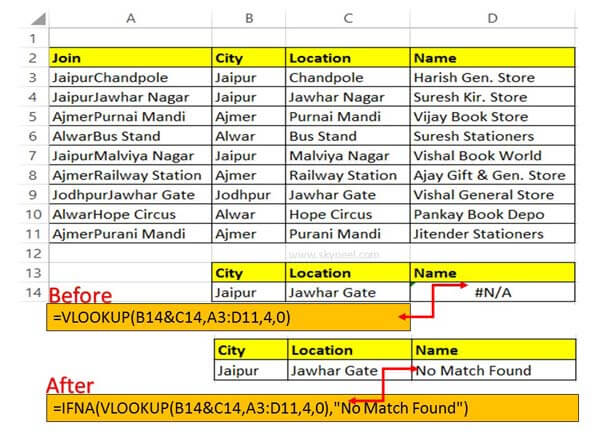# How to search value using VLookup with IFNA function in Excel

During search value using VLookup you can view #N/A error value. Now during searching you can use IFNA with VLookup function in Excel to solve the issue.

Functions play an important role during searching the value. But sometime during searching the value you can view #N/A error value in active sheet. Excel allows you to quickly search value using VLookup with IFNA function in Excel to resolve the #N/A error issue.

During searching any specific values in a large worksheet data it is not possible you will get always accurate result. Sometime you can get error value after applying the VLookup function during searching the value in active worksheet. Excel provide different function to resolve your your problem which you face during searching the value or resolve error value.

## How to search value using VLookup with IFNA function in Excel

When you try to search value using VLookup function sometime you will get an error value. That type of error value generated when your lookup value does not match with the given table array. In that situation if you don’t want to view #N/A error value and show blank or an error message then you can use IFNA function in Excel.For eg: As per the given table, If you try to search city name and location with the help of VLookup function. If your lookup values does not match then you will get #N/A error value. In that situation you must have to use IFNA with VLookup function in active worksheet. This function allows you to resolve the error value and shows any blank or other message in-front of you.

Before start the process how to search value using VLookup with IFNA function in Excel, first you must know complete information about them, have a look.

### VLookup function in Excel

VLookup is an important lookup function which is found in Lookup & Reference category. It allows you to searches a value in the extreme left column of the table_array and provides the value in the same row which is based on the index number.

Syntax of VLookup function:

VLookup (lookup_value, table_array, col_index_num, [range])

Note: Before using the VLookup function, first you need to arrange first column of a table or array in the ascending order, otherwise VLookup function is not able to provide the accurate result.

Lookup_value: The value to be searched in the extreme first column of the table array.

Table_array: Two or more columns of data. Give cell reference of a range / range name.

Col_index_num: The column index_number in the table from which the searched value must be returned.

If col_index_num of returns 1 the value in the first column in table_array. If col_index_num is: Less than 1, this function returns an error value the #VALUE!. Sometime it is a greater than the number of columns in table_array, this function returns error value #REF!.

Range_lookup: It is an options part of this function. There are two options available in this part.

TRUE: Appropriate Match: This option work properly when first column of your table will be sorted in ascending order only otherwise it displays wrong result.

False: Exact Match: VLookup by default check only on exact match method.

### IFNA Function in Excel

IFNA function returns the value which you specify, If the formula returns the #N/A error value; otherwise returns the result of the formula.

Syntax of IFNA function:

IFNA(value, value_if_na)

The IFNA function syntax has the following arguments.

Value (Required): The argument that is checked for the #N/A error value.
Value_if_na (Required): The value to return if the formula evaluates to the #N/A error value.

Step 1: Prepare the following given worksheet to search the value and resolve the #N/A error value.

Step 2: Apply the following given formula in cell D14 =VLOOKUP(B14&C14,A3:D11,4,0) and press enter. You can check given if, city and location does not match in the given criteria then you can view #N/A error value.

Step 3: To resolve that issue you must have to edit the formula in cell D14 =IFNA(VLOOKUP(B14&C14,A3:D11,4,0),”No Match Found”) and press enter.

I hope after reading this guide you can easily search value using VLookup and resolve #N/A error value with IFNA function in Excel. If you have any suggestion regarding this guide then please write us in the comment box. Thanks to all.